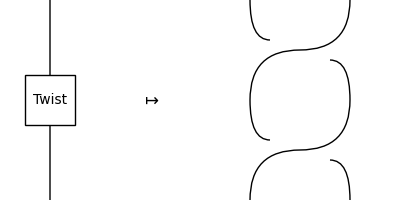# Diagram#

class discopy.ribbon.Diagram(inside, dom, cod, _scan=True)[source]#

A ribbon diagram is a pivotal diagram and a balanced diagram.

Parameters:
• inside (Layer) – The layers of the diagram.

• dom (pivotal.Ty) – The domain of the diagram, i.e. its input.

• cod (pivotal.Ty) – The codomain of the diagram, i.e. its output.

trace(n=1, left=False)[source]#

The trace of a ribbon diagram.

Parameters:

n – The number of wires to trace.

cup(x, y)[source]#

Post-compose a ribbon diagram with a cup between wires `i` and `j` by introducing braids.

Parameters:
• i – The wire on the left of the cup.

• j – The wire on the right of the cup.

to_ribbons()[source]#

Doubles evry object and sends the twist to the braid.

Example

```>>> x = Ty('x')
>>> braided_twist = Diagram.twist(x).to_ribbons()
```braid_factory#

alias of `Braid`

cap_factory#

alias of `Cap`

cup_factory#

alias of `Cup`

factory#

alias of `Diagram`

twist_factory#

alias of `Twist`